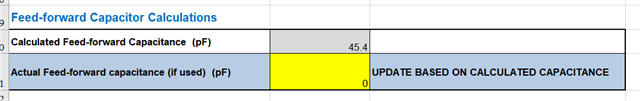If you have a related question, please click the "Ask a related question" button in the top right corner. The newly created question will be automatically linked to this question.

# LMR33630-Q1: LMR33630_calculator_A0 feed-forward cap calculate

Part Number: LMR33630-Q1
Other Parts Discussed in Thread: LMR33630

Hi, Team

My customer is using our "LMR33630_calculator_A0" for circuit design recently and they have a question with our LMR33630_calculator_A0.

For Feed-forward Cap calculator, the calculator allows user to fill in the actual capacitance value of the capacitor, and then the theoretical value of the capacitance will be calculated. This logic makes me confused.1. Why does this calculator ask me to fill in the actual cap value used?
In my opinion, the correct logic is: I fill in other parameters, and the calculator calculates the theoretical cap value according to my needs.

2. When I fill in the actual used cap value, the calculated value changes.
Why is the calculated cap value affected by the actual used cap value?

I think these two values belong to the same capacitor.